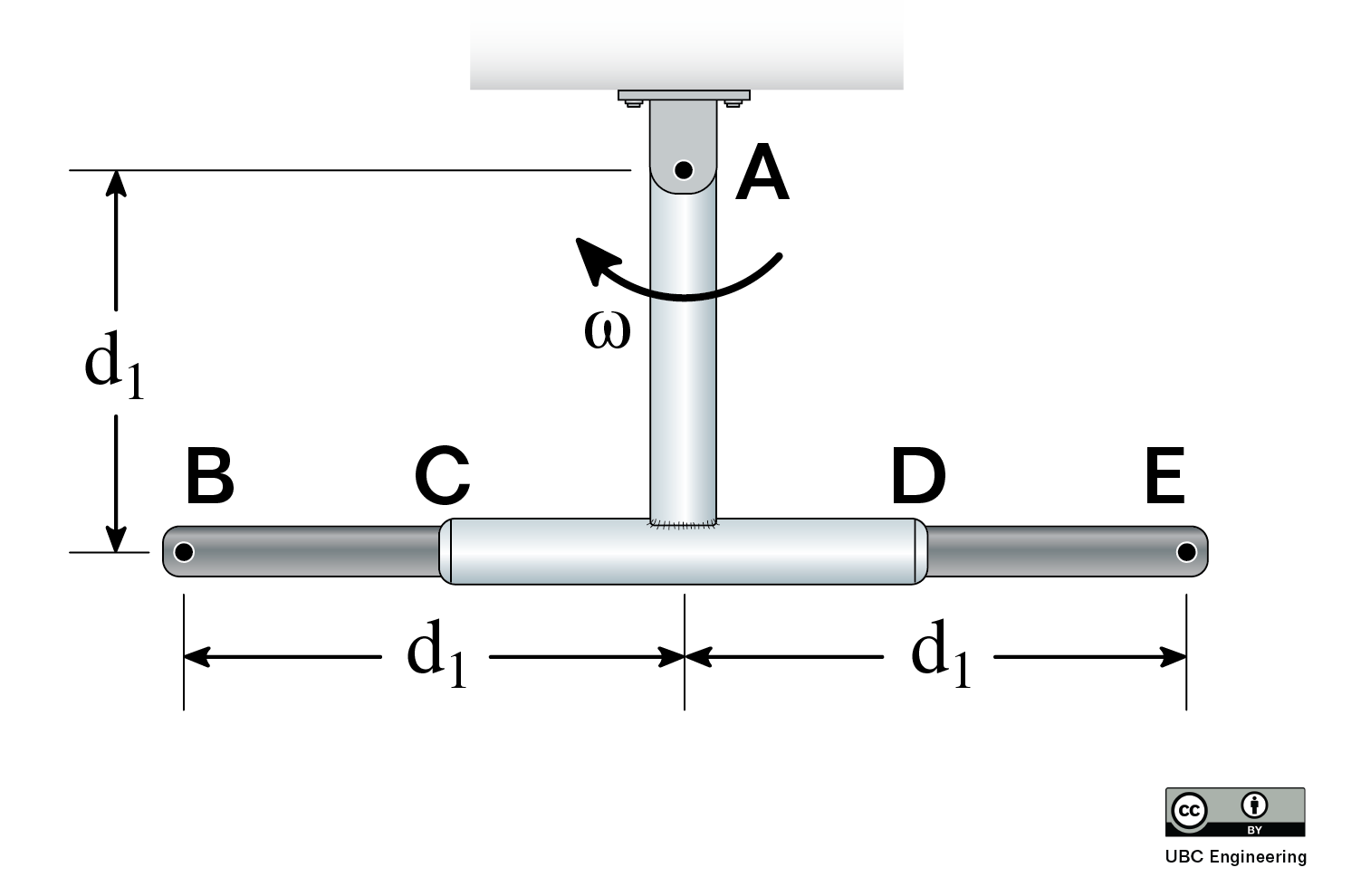﻿ Mechanics Map - Rotating Frame Analysis

# Rotating Frame Analysis

This section presents relative motion using vector notation.

Rotating frame analysis is a specialized part of relative motion analysis. It is typically performed in Cartesian (x-y) coordinates for rigid bodies. In the previous section, rotating frames in polar coordinates were used to solve problems with formulas similar to those in particle kinematics. We will adopt a slightly different notation that is specialized to rigid bodies. These formulas are the most general planar kinematics formulas - that is, they can always be used, and will provide the correct answer. But they are unnecessarily complicated for many types of motion, such as pure rotation.

Rotating frame analysis is really important for cases where objects are not pinned to each other. When do you use rotating frames? When one object is sliding against another object, or two objects are not even in contact, but you want to know something about the motion relationship between them and/or are given information about the motion relationship between them.

## Reference Frames

In most of the preceding material, you have worked with the fixed reference frame, Oxyz, and a translation (but not rotating) reference frame attached to the rigid body at a point A, frame Ax'y'z'.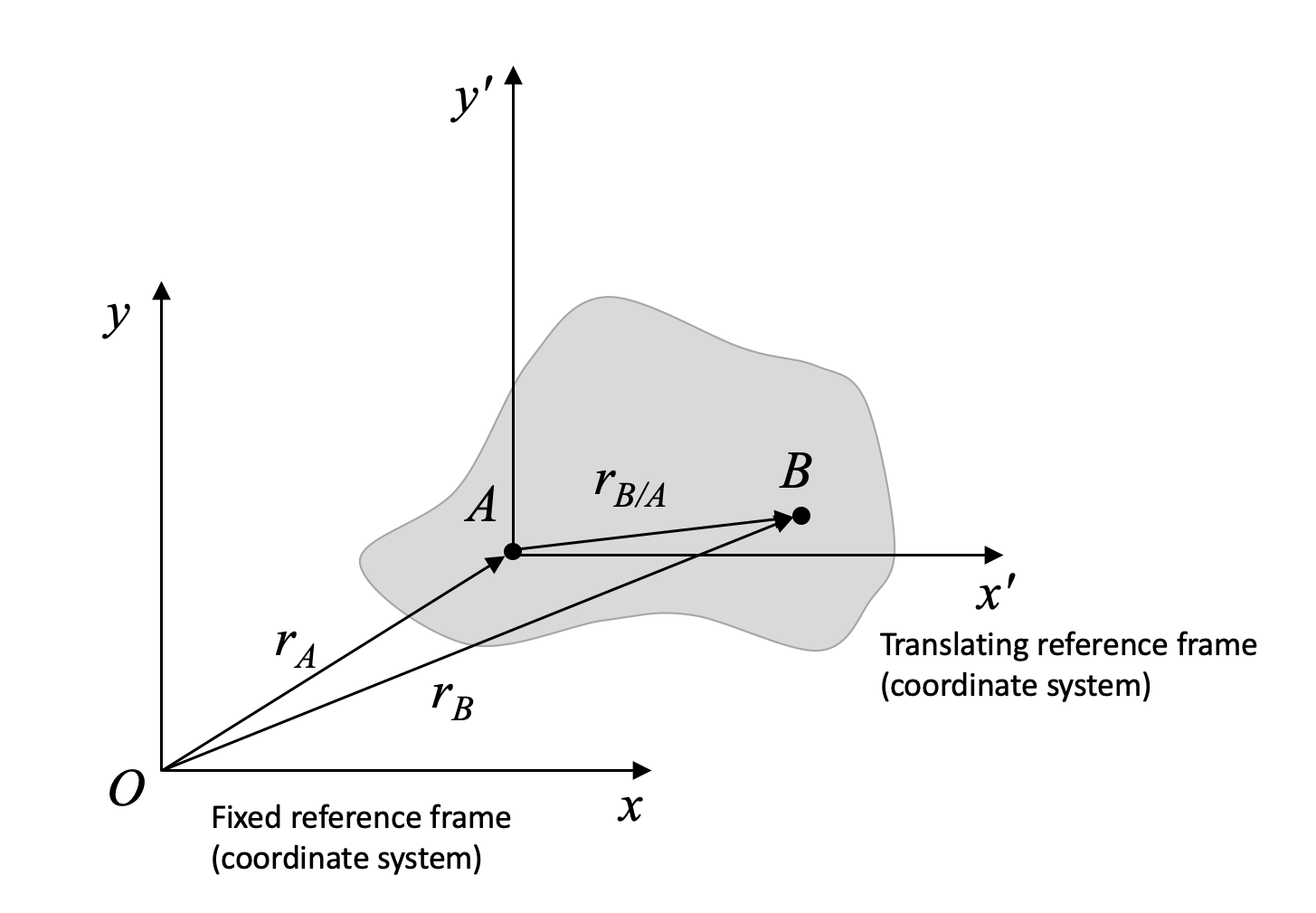A rigid body with a translating (not rotating) reference frame, x'y'z', attached at point A, relative to the fixed frame, xyz, at O.

This has given us relative motion equations:

Position: $\vec{r}_{B/O}=\vec{r}_{A/O}+\vec{r}_{B/A}$ $\vec{v}_{B/O}=\vec{v}_{A/O}+\vec{r}_{B/A} = \vec{v}_{A/O}+ \vec{\omega} \times \vec{v_{B/A}}$ $\vec{a}_{B/O}=\vec{a}_{A/O}+\vec{a}_{B/A}= \vec{a_{A/O}} + \vec{\alpha} \times \vec{a_{B/A}} - \omega^2 \vec{r_{B/A}}$

Note that the notation rB/O, where O is the fixed frame, can also be written rB. Both indicate an absolute position (or velocity or acceleration) - that is, a value with respect to the fixed frame, O.

## Rotating Frames

Now, we will consider a reference frame that is attached to a point on the rigid body and both rotating and translating with the rigid body. We will introduce some extra terms to account for the rotation of the frame.

Consider a rigid body with frame x'y'z' at point A. x'y'z' translates and rotates with the body. A bug, B, is crawling along the body.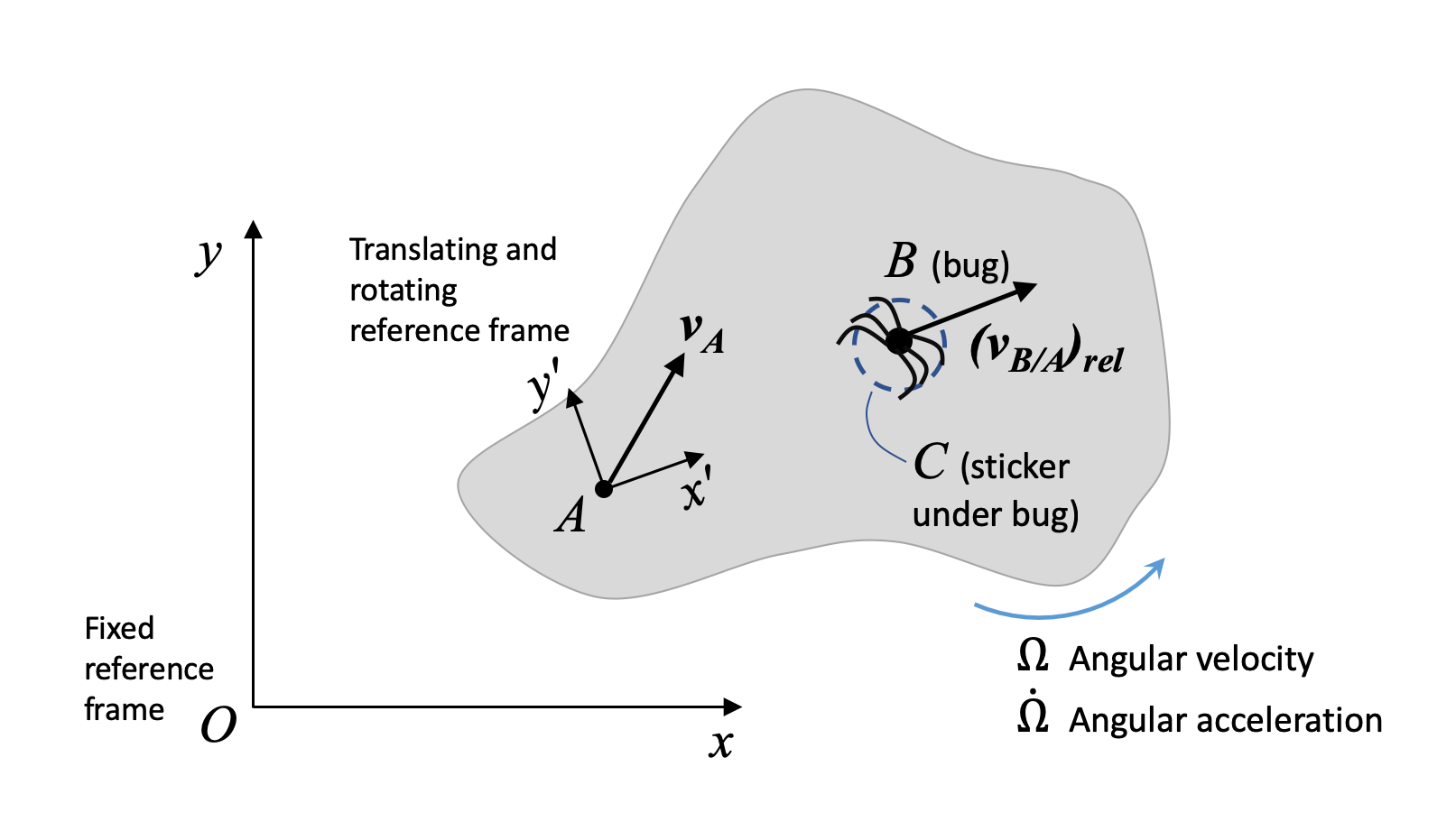A rigid body with a translating AND rotating reference frame, x'y'z', attached at point A. A bug, B, is crawling along the rigid body, with a velocity relative to the rotating frame. The bug is currently above a sticker, C, that is rigidly fixed to the rigid body.

For an observer sitting at A, the bug appears to be crawling away in a straight line. But for an observer sitting at O, the bug does not look like it is moving in a straight line, because the body it is crawling on is also moving (translating and rotating). We would like to describe the motion of the bug as seen by an observer at O. To remind ourselves that we are dealing with a rotating frame attached to one body, we use capital omega (Ω) to denote the angular velocity of the object that the rotating frame is attached to, and omega dot for the angular acceleration of the object with the rotating frame attached. Finally, we use brackets and the subscript "rel" to denote values of velocity or acceleration expressed with respect to the rotating frame.

Recall that vectors have both magnitude and direction. We can express a vector in components with respect to the i and j unit vectors:

 $\overrightarrow{r}_{B/O}=r_{B/O, x} \hat{i}+r_{B/O, y} \hat{j}$

Normally, when we take a time derivative of such an expression, the length of the vector (i.e. the rB/O, x and rB/O, y terms) change, but the unit vectors do not. In the case of the rotating frame, the unit vectors change. Now, they don't change in length - they remain unit vectors. But they are attached to a rotating body, which means they also rotate. That is, their directions change, and we have to account for that when deriving velocity and acceleration expressions.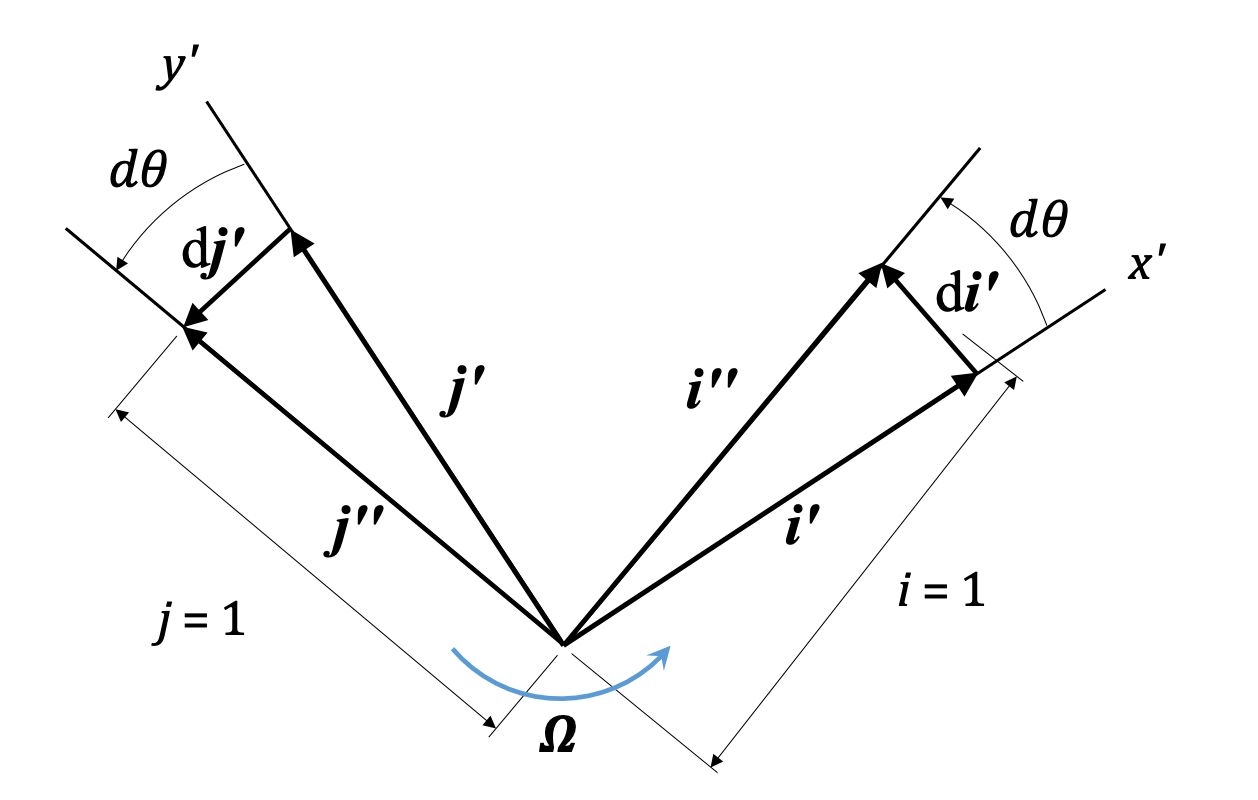When the reference frame rotates with the rotating rigid body, the unit vectors change with time.

You can see we get small vectors di' and dj' that describe the change in direction of the unit vectors, and the magnitude of that change is directly related to Ω, the angular velocity of the rigid body and the rotating frame.

 $\frac{d\hat{i'}}{dt} = \Omega \hat{k} \times \hat{i'} = \Omega \hat{j'}$ $\frac{d\hat{j'}}{dt} = \Omega \hat{k} \times \hat{j'} = -\Omega \hat{i'}$

When we differentiate the relative position of the bug, B, with respect to the rotating frame, A, we have:

 $\frac{d\vec{r}_{B/A}}{dt} = (\dot{r}_{B/A,x'} \,\hat{i'}+ \dot{r}_{B/A,y'}\,\hat{j'}) + \vec{\Omega} \times ({r_{B/A,x'}}\,\hat{i'}+ {r_{B/A,y'}}\,\hat{j'})$
 New term: $(\dot{r}_{B/A,x'} \,\hat{i'}+ \dot{r}_{B/A,y'} \,\hat{j'}) = (\vec{v}_{B/A})_{rel}$ $\vec{\Omega} \times ({r_{B/A,x'}} \,\hat{i'} + {r_{B/A,y'}} \,\hat{j'}) = \vec{\Omega} \times \vec{r}_{B/A}$

The new term above describes the motion of the bug due to the rotation of the rigid body (and the x'y'z' frame), while the familiar term above describes the motion of the bug as seen by an observer fixed to x'y'z'. Our final expression for the velocity of the bug with respect to the fixed frame is:

 Rotating frames velocity: $\mathbf{\vec{v}_{B/O} = \vec{v}_{A/O} + \vec{\Omega} \times \vec{r}_{B/A} + (\vec{v}_{B/A})_{rel}}$

This final expression has the new term, (vB/A)rel, which describes the motion of the bug as viewed from the rotating frame. You can note that if the bug is not moving, (vB/A)rel = 0, and we get the familiar expression from translating frames. Thus, this expression is the most general relative velocity expression.

We can similarly derive an acceleration equation by differentiating the above velocity equation. The final expression is:

Rotating frames acceleration: $\mathbf{\vec{a}_{B/O} = \vec{a}_{A/O} + \dot{\vec{\Omega}} \times \vec{r}_{B/A} }$ $\mathbf{+ \, \vec{\Omega} \times (\vec{\Omega} \times \vec{r}_{B/A}) + 2 \vec{\Omega} \times (\vec{v}_{B/A})_{rel} + (\vec{a}_{B/A})_{rel} }$
 Motion of rotating frame attached to rigid body: $\vec{a}_{A/O} + \dot{\vec{\Omega}} \times \vec{r}_{B/A} + \vec{\Omega} \times (\vec{\Omega} \times \vec{r}_{B/A})$ $2 \vec{\Omega} \times (\vec{v}_{B/A})_{rel}$ $(\vec{a}_{B/A})_{rel}$

Note that, for planar (2D) motion:

 $\vec{\Omega} \times (\vec{\Omega} \times \vec{r}_{B/A}) = -\Omega^2 \vec{r}_{B/A}$

Again, if the bug is sitting still, both "rel" terms become zero (it is not moving wrt the rigid body), and we are left with the same relative motion expression used with translating frames. So, you can always use this expression.

When tackling these problems, there are several important things to remember:

1. You need to pick the correct body to attach the rotating frame to. The correct body will have another body sliding against it. If the object of interest is pinned to the body you chose, it might not be correct.
2. It is often helpful to align your rotating frame such that you end up with a simplified "rel" term or terms. For example, line up the x' axis with the direction of movement of the bug, so that (vB/A)rel is only in the x'-direction.
3. The components of all terms of any one equation must be computed along the same i and j directions. If the frames are not aligned, you must pick ONE FRAME and express all terms along that the directions of the ONE FRAME. (You may be able to switch between frames in different equations, but make sure you are careful with notation!)
4. When you are given information in a problem, pay careful attention to what frame is being referred to.
5. Make sure you include all the terms in the acceleration equation! It's easy to miss one. You should have five terms.

These are some of the most complex problems in planar kinematics, so take your time!

### Planar rotating frames equations

 Velocity: $\mathbf{\vec{v}_{B/O} = \vec{v}_{A/O} + \vec{\Omega} \times \vec{r}_{B/A} + (\vec{v}_{B/A})_{rel}}$ $\mathbf{\vec{a}_{B/O} = \vec{a}_{A/O} + \dot{\vec{\Omega}} \times \vec{r}_{B/A} }$ $\mathbf{ - \, \Omega^2 \vec{r}_{B/A} + 2 \vec{\Omega} \times (\vec{v}_{B/A})_{rel} + (\vec{a}_{B/A})_{rel} }$

## Worked Problems:

### Question 1:

A collar which is pinned to rod CD slides along rod AB. At the instant shown, when ϕ=60 deg, the angular velocity of rod AB is 2 rads/s in the direction shown. Find the rate at which C travels along rod AB, and the angular velocity of rod CD. Assume d1 = 0.3 m and d2 = 0.2 m.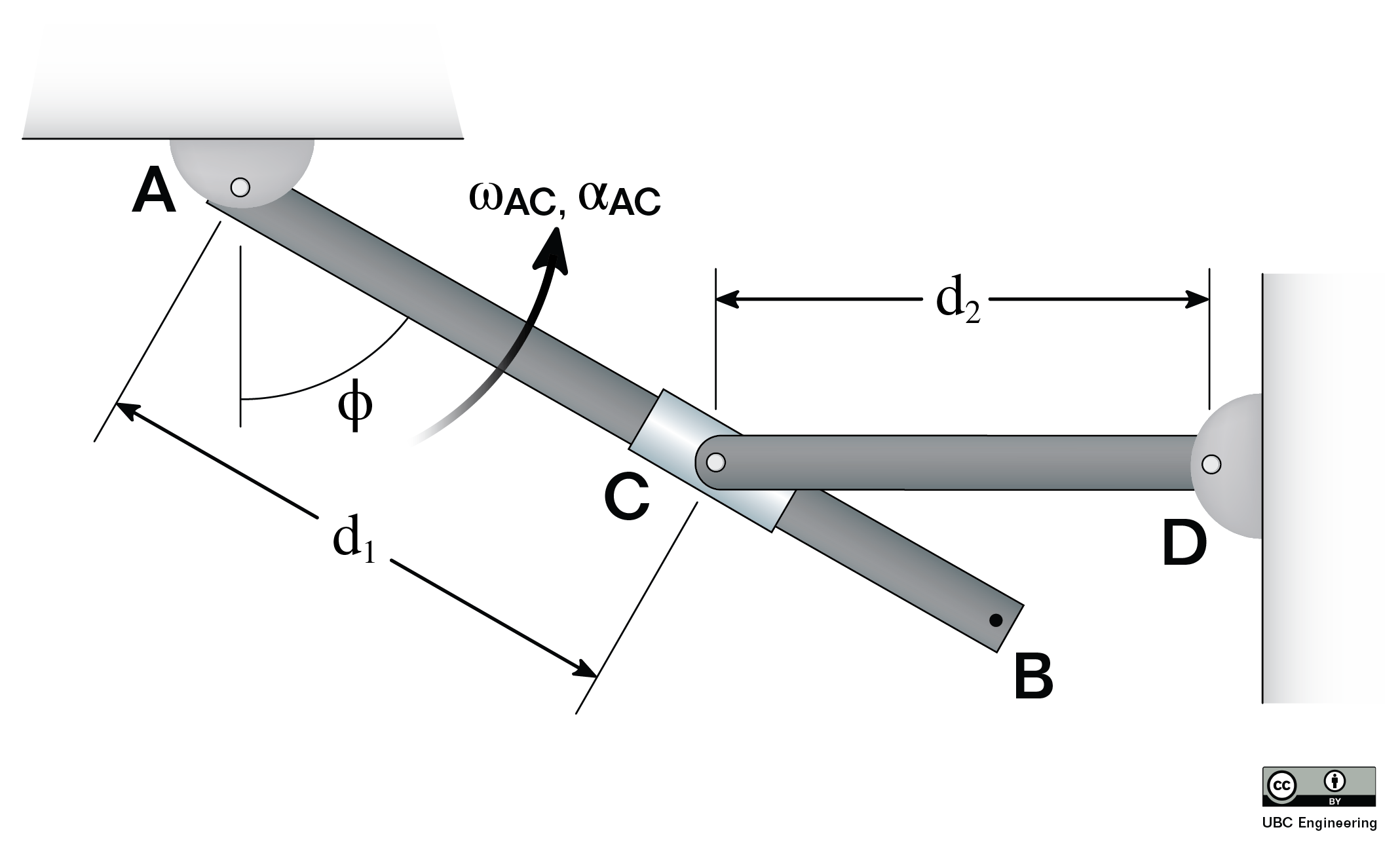### Question 2:

A camera drone, D, flies over a car race in a curved trajectory (center O) with a constant ground-speed velocity of vD = 9 m/s. At the moment shown, car C is traveling with velocity of vC = 12 m/s and an acceleration of aC = 2 m/s2 as shown. Assume d1 = 7.5 m, d2 = 3 m.

a) Find the velocity of the car as observed by the camera on drone D at this instant.

b) Find the acceleration of the car as observed by the camera on drone D at this instant.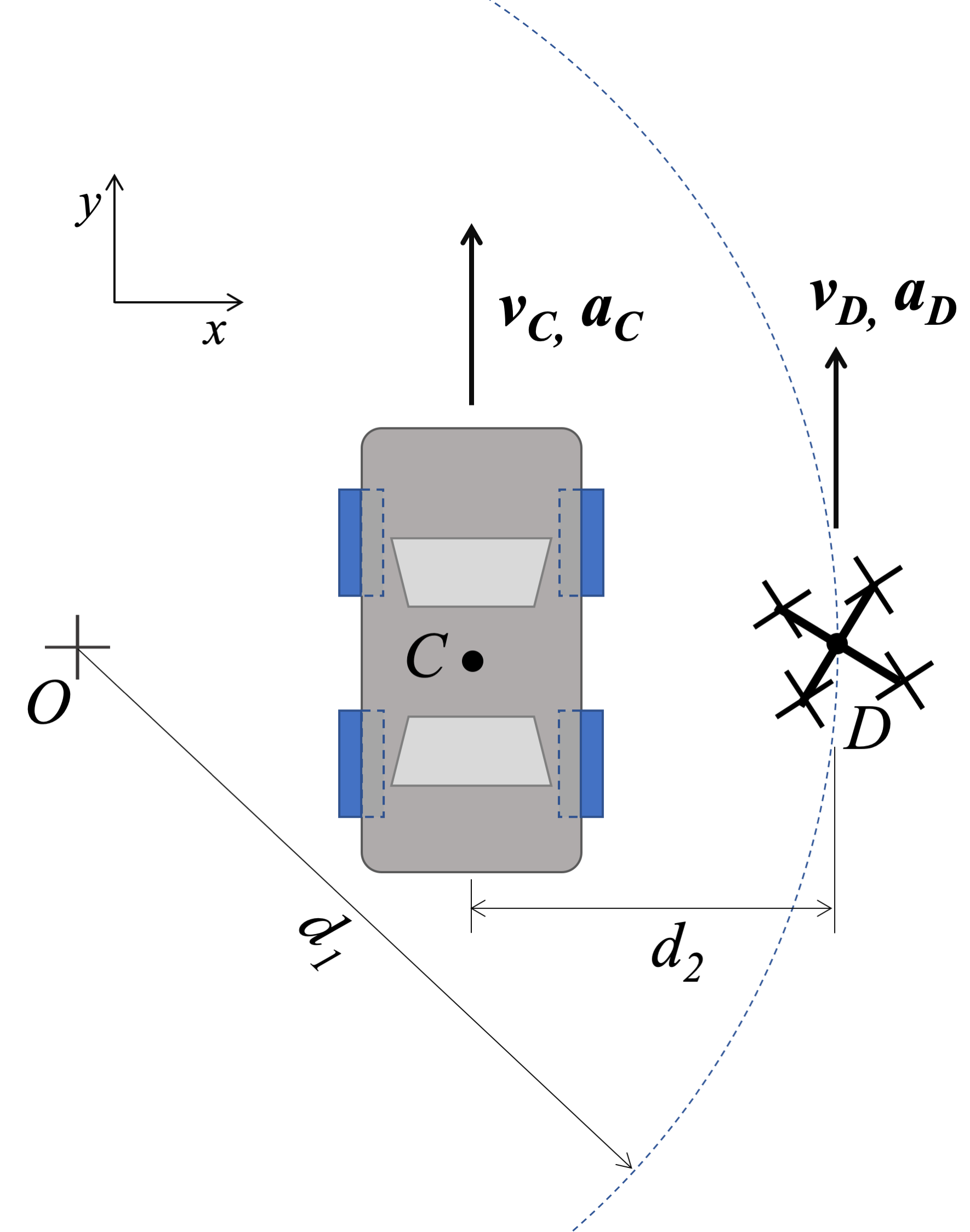### Question 3:

A breathing exercise video graphic (somewhat similar to this one) shows a small circle moving in a constrained circular path (constant radius 80 cm) at a constant angular velocity of 0.4 rad/s around an expanding and contracting inner circle. The inner circle expands and contracts sinusoidally, from a minimum radius of 30 cm to a maximum radius of 60 cm. The distance from the center of the inner circle to a point on the edge of the inner circle can be described by the equation r=0.45-0.15 sin⁡θ (in m), where θ is the position of the small circle (zero at x-axis).

Find the velocity and acceleration of point B on the edge of the inner circle as viewed by an observer on the small circle at point A (Fig. (c)). θ=45°, α=45°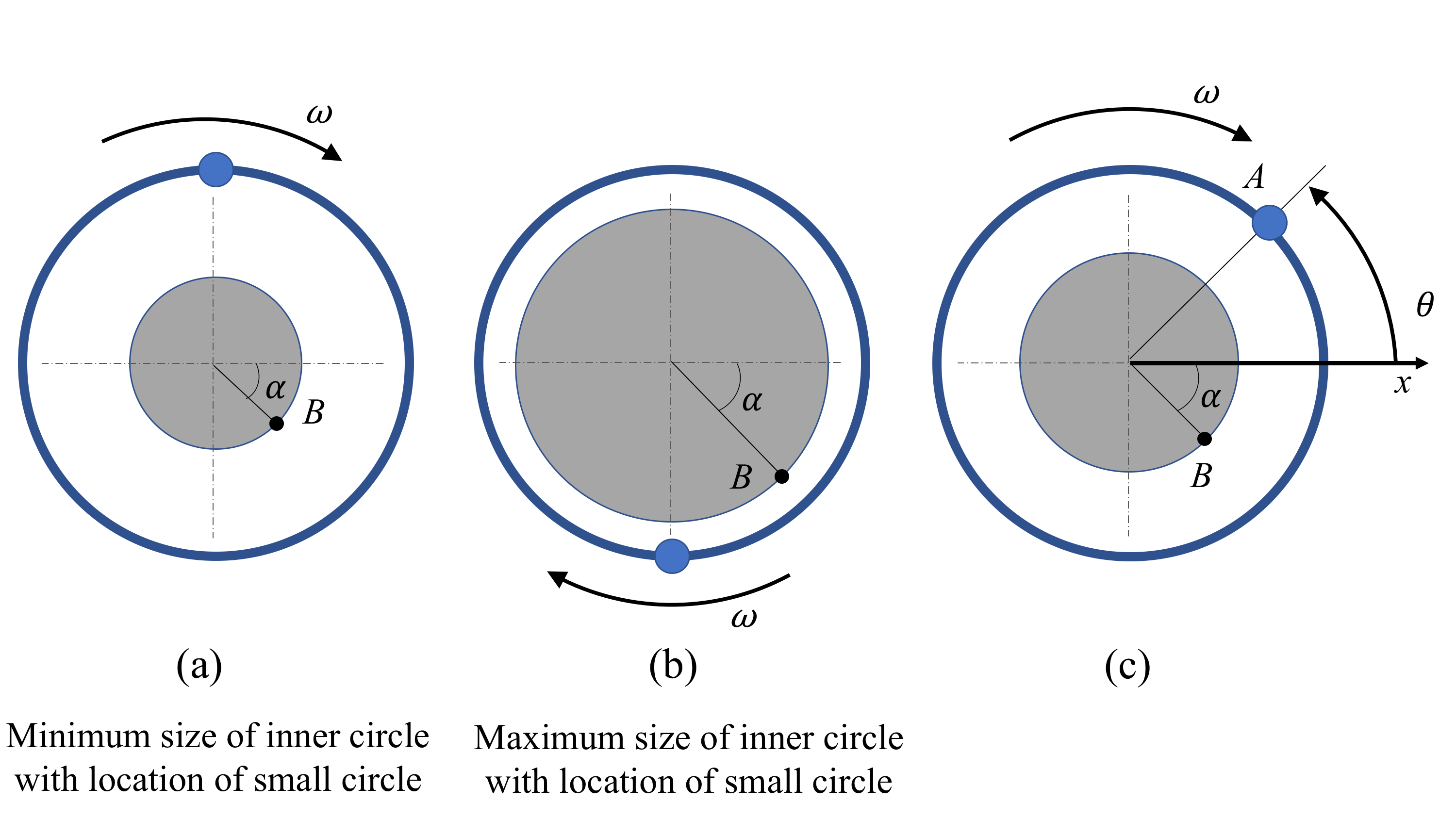### Question 4:

The crank-rocker mechanism as shown below consists of a crank with a radius of .5 meters rotating about its fixed center at C at a constant rate of 2 rad/s clockwise. Rocker AB fixed at it's base at A and connects to point B along the edge of the crank. The pin at point B can slide along a frictionless slot in AB. In the current state, what is the angular velocity of rocker AB?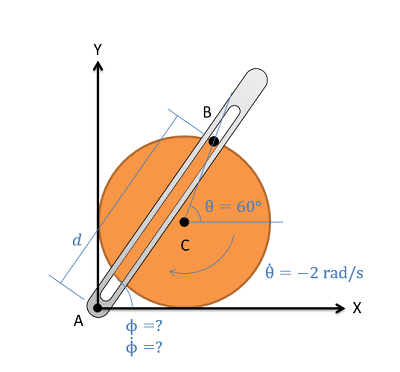### Question 5:

A very funny engineer has attached her prank punching machine to a robotic arm. If the boxing glove moves at a maximum 1m/s constant velocity, relative to the link CD, what is the velocity and acceleration of the glove at the instant shown? The lengths of the linkage arms are given as rAB=0.3 m, rBC=0.6 m and rCD=0.4 m. The angles are given as θ=30 deg, φ=50 deg and γ=15 deg. Arm BC is rotating at ωBC=2 rad/s and αBC=1 rad/s^2, while arm CD is rotating at ωCD=-0.25 rad/s and αCD=0.25 rad/s2. Arm AB is stationary throughout.### Question 6:

Boat A is traveling forward (in positive y) with a velocity of 25 m/s and an acceleration of 4 m/s2. The person in dingy B is traveling in a circle (as they only have one oar). They have a forward (in positive y) velocity of 5 m/s and acceleration of -1 m/s2 (as they have lost focus while watching boat A). The radius of dingy B’s path is r = 20 m, and the distance between the vessels is d = 10 m.

Find the velocity and acceleration of boat A as seen by the occupants of dingy B.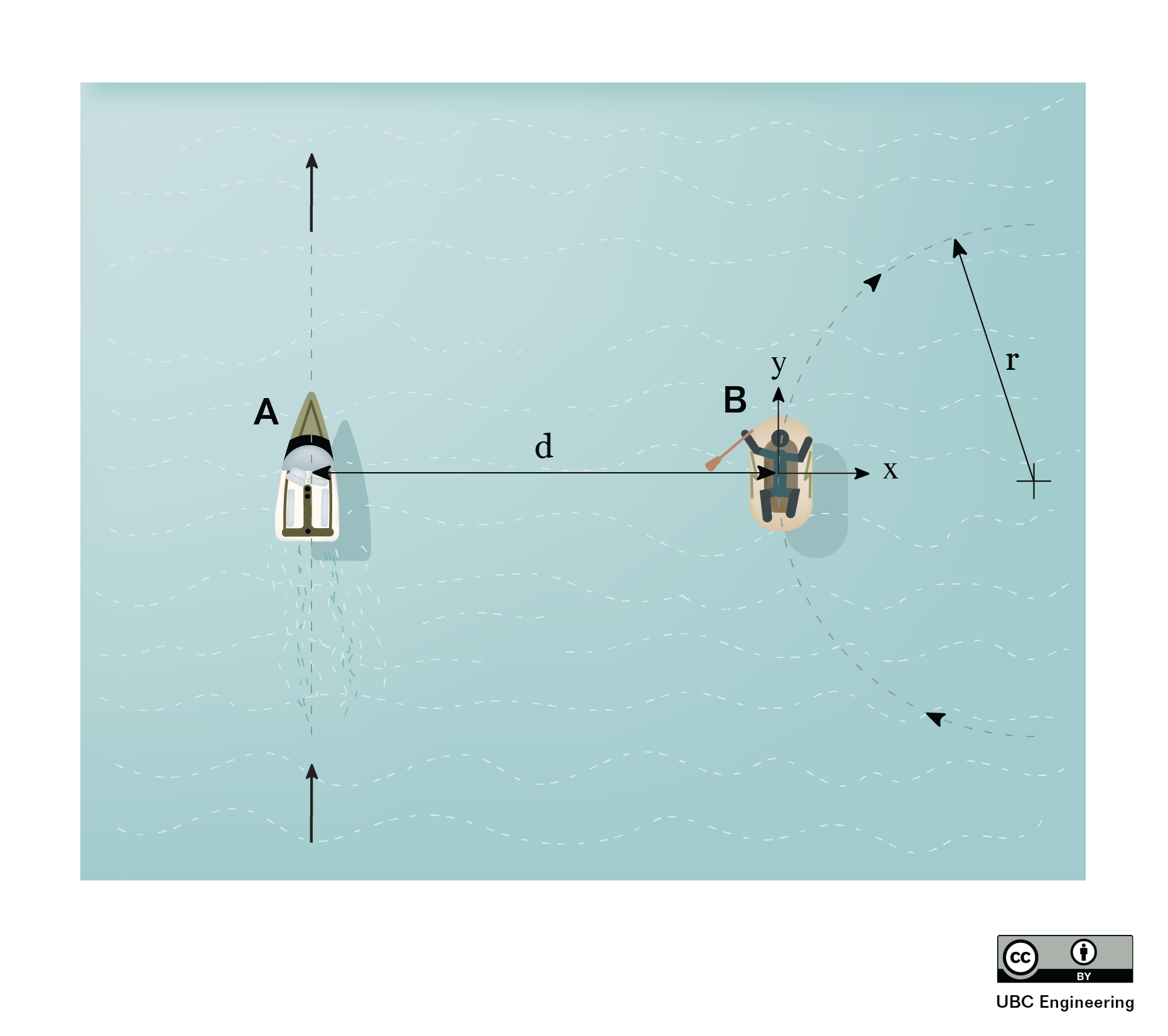### Question 7:

Boat A is traveling forward (in positive y) with a velocity of 25 m/s and an acceleration of 4 m/s2. The person in dingy B is traveling in a circle (as they only have one oar). They have a forward (in positive y) velocity of 5 m/s and acceleration of -1 m/s2 (as they have lost focus while watching boat A). The radius of dingy B’s path is r = 20 m, and the distance between the vessels is d = 10 m.

Find the velocity and acceleration of dingy B as seen by the occupants of boat A.### Question 8:

Body ACD is pinned at A and is rotating with an angular velocity of ω = 3 rad/s as shown at this instant. Bar BE slides through tube CD, such that, at this instant, the velocity of B is purely in the y-direction, and the acceleration of B is zero (as observed from the fixed frame). Find the vectors for the velocity and acceleration of B in the rotating frame, (vB/A)x'y'z' and (aB/A)x'y'z', and the angular acceleration of ACD at this instant. Distance d1 = 0.5 m.